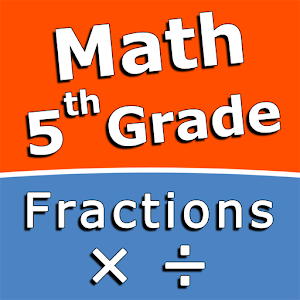English

Every day we offer FREE licensed Android apps and games you’d have to buy otherwise.\$2.49
EXPIRED

# Android Giveaway of the Day - Multiply and divide fractions - 5th grade math

Multiply and divide fractions!
\$2.49 EXPIRED
User rating: 0 (0%) 0 (0%)

This giveaway offer has expired. Multiply and divide fractions - 5th grade math is now available on the regular basis.

Intuitive interface powered by handwriting input and a choice of three fun and engaging mini games in addition to a regular math trainer mode make our app stand out from the crowd of generic math learning apps.

With Multiply and divide fractions - 5th grade math you can practice and improve the following math skills:
- Multiply fractions by whole numbers
- Multiply two fractions
- Multiply a mixed number by a whole number
- Multiply a mixed number by a fraction
- Multiply two mixed numbers
- Divide fractions by whole numbers
- Divide whole numbers by fractions
- Divide two fractions
- Divide fractions and mixed numbers

Choose between four different modes:
- Math Trainer
- Math Shot mini-game
- Math Gravity mini-game
- Math Trick Shot mini-game

Sergey Malugin

Education

1.0.0

11M

Everyone

4.0 and up

## Comments on Multiply and divide fractions - 5th grade math

Thank you for voting!
Please add a comment explaining the reason behind your vote.
Windows Giveaway of the Day\$14.99
free today
Update your registry and optimize the performance of your PC!